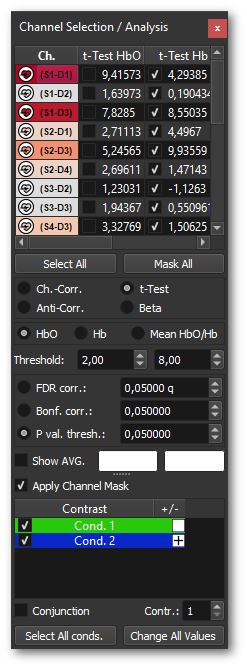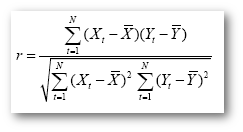Channel Selection and Analysis Window# Channel Selection and Analysis WindowThe channel selection and analysis window contains a table of the available channels, including different statistical measures. The checkbox on the left side of the t-Test HbO column shows or hides this channel in the selected channels plot. The checkbox on the left side of the t-Test Hb column shows or hides this channel in the all channels plot. There are two convenient buttons to toggle the selection and masking of all channels at the same time. Press the “Select All” button (below the table) to select / deselect all channels. Use the “Mask All” push button to toggle the masking for all channels. A little heartbeat icon is added to the left of the channel selection table when a heartbeat is detected. Please check the general linear model section for more details about the heartbeat detection algorithm.

### Statistics input

The radio buttons Ch.-Corr., Anti-Corr., Beta, t-Test toggle the statistical output in all views showing statistical values.

### Ch.-Corr.

The channel correlation option allows to inspect the correlation of one channel to all other channels for either HbO or Hb. The correlation is based on the Pearson correlation coefficient r:Index t runs over time points (t for “time”) identifying pairs of temporally corresponding values from the reference (Xt) and data (Yt) time courses. In the numerator, the mean of the reference and data time course is subtracted from the respective value of each data pair and the two differences are multiplied. The resulting value is the sum of these cross products, which will be high if the two-time courses covary, i.e. if the values of a pair are both often above or below their respective mean. The term in the denominator normalizes the covariation term in the numerator so that the correlation coefficient lies in a range of -1 and +1. A value of +1 indicates that the reference time course and the data time course go up and down in exactly the same way, while a value of -1 indicates that the two-time courses run in opposite directions. A correlation value close to 0 indicates that the two-time courses do not covary, i.e. the value in one-time course cannot be used to predict the corresponding value in the other time course.

If the Show AVG. option is selected, the Ch.-Corr. is performed based on this average time course of the selected channels.

### Anti-Corr.

The anti-correlation option is based on the Pearson correlation as well, but it calculates the correlation between HbO and Hb of each channel individually. All three columns (HbO, Hb, Mean HbO/Hb) will display the same value for a given channel. The anti-corr. option allows to check if the assumption of an anti-correlation between HbO and Hb is valid. This is especially important for the CBSI motion correction, which heavily relies on the validity of this assumption!

### Beta

The beta option will display the beta estimate of each channel based on the selected contrast in the contrast window (bottom of this window). Here the “+” and “-” symbols indicate the sign of the beta. If multiple conditions are checked using either the “+” or the “-” symbol, the average of the betas will be calculated and displayed. This allows, for example, to show differences in signal amplitude across different conditions.

Note: The Conjunction checkbox has no effect on the beta calculations! ### t-Test ### The t-test option follows the same logic as the beta option, except that the displayed values are t-values.

## Signal source

The radio button HbO, Hb, Mean HbO/Hb toggles the color coding as well as the spectrogram to color code the contrast of the selected radio button using either HbO, Hb or the mean as signal source. Hereby, the mean is the average of the HbO and Hb results and not based on the averaged time courses of HbO and Hb!

## Thresholding

The color coding of the row headers and layout views can be adapted using the Threshold spin boxes. Minimum threshold is encoded as blue and it gradually fades into red, representing maximum t-threshold, as shown in the color bar on the right. The min and max thresholds can be defined using the threshold spin boxes (left min, right max) below the tables.

## Multiple Comparison Correction

Different correction methods for the t-statistics are offered below the threshold options. These settings do not apply to Ch.-Corr., Anti-Corr. and Beta. More details about the different procedures are describe in the Multiple Comparisons Problem section at the bottom of this page.

## Average Signal

In many experiments it can be useful to inspect the average of certain channels to decide which sources of the signal can reliably be used for, for example, neurofeedback. The average of the selected channels (t-Test HbO column) is shown in the selected plot using a black color. The Show avg. checkbox has three states: Not checked, meaning no averaging, partially selected, showing the black averaged signal together with the selected channels, and fully checked, which only shows the black average signal. On the right to the checkbox, one can find the corresponding statistics (based on the selected criteria for HbO and Hb of the averages).

The Apply Channel Mask option is only available in the Simulated Mode. This option allows to show only the masked channels defined in the loaded project.

## Contrasts

A table containing the available contrasts based on the provided protocols or triggers can be found at the bottom of the window. The value column defines the contrast parameter used in the t-Test of the HbO and Hb data. The checkbox left to the contrast name allows to show or hide conditions in the event related averaging plot. More details about the contrast dialog can be found in the contrasts section.

# The Multiple Comparisons Problem

### False Discovery Rate

The (FDR corr.) checkbox applies the so-called false discovery rate correction using a given q value, defined in the corresponding spin box. One can find more information about multiple comparison correction in the following article:

Archana K. Singh, Ippeita Dan, Exploring the false discovery rate in multichannel NIRS. 2006.

While the described multiple comparison correction methods aim to control the family-wise error rate, the false discovery rate (FDR) approach (Benjamini & Hochberg, 1995) uses a different statistical logic, and has been proposed for fMRI analysis by Genovese and colleagues (2002) and fNIRS by Archana and colleages (2006). In this approach, the number of false positive channels among the subset of channels labelled as significant is controlled, instead of the overall number of false positive channels. Supra-threshold channels with a specified threshold value are called “discovered” channels or “channels declared as active”. With a false discovery rate of q < 0.05, one would accept that 5% of the discovered (supra-threshold) channels would be false positives. Given a desired false discovery rate, the FDR algorithm calculates a single-channel threshold, which ensures that channels beyond this threshold do not contain more than the specified proportion of false positives. A q value of 0.05 also means that one can “trust” 95% of the supra-threshold (i.e. color-coded) channels, since the null hypothesis has been rejected correctly. Since the FDR logic relates the number of false positives to the amount of truly active channels, the FDR method adapts to the amount of activity in the data; The method is very strict if there is not much activity in the data but assumes less conservative thresholds when larger regions of the brain show task-related effects. In the extreme case, when not a single channel is truly active, the calculated single-channel threshold is identical to the one computed with the Bonferroni method. The FDR method appears ideal for fMRI data because it does not require spatial smoothing and it detects channels with a high sensitivity (low beta error), if there are true effects in the data.

### Bonferroni Correction

The Bonferroni correction method is a simple multiple comparison correction approach adjusting the single-channel threshold in such a way that we retain an error probability of 0.05 at the global level. With N independent tests, this is achieved by using a statistical significance level, which is N times smaller than usual. The Bonferroni correction can be derived mathematically as follows. Under the assumption of independent tests, the probability that all of N performed tests lead to a sub-threshold result is (1-p)N and the probability to obtain one or more false positive results is 1-(1-p)N. In order to guarantee a global or family-wise error (FWE) probability of pFWE = 1-(1-p)N, the threshold for a single test, p, has to be adjusted as follows: p = 1-(1-pFWE)1/N. For small pFWE values (e.g. 0.05), this equation can be approximated by p = pFWE / N. This means that, in order to obtain a global error probability of pFWE < 0.05, the significance level for a single test is obtained by dividing the family-wise error probability by the number of independent tests. Given 100,000 channels, we would obtain an adjusted single-channel threshold of pv = pFWE / N = 0.05/100000 = 0.0000005. The Bonferroni correction method guarantees that we wrongly accept even a single channel as significant with an error probability of only 0.05. The method, thus, controls the alpha error across all channels, and it is therefore called a family-wise correction approach. For fMRI data, the Bonferroni method would be a valid approach to correct the alpha error, if the data at neighbouring channels was truly independent from each other. Neighbouring channels, however, show similar response patterns within functionally defined brain regions, such as the fusiform face area (FFA). In the presence of such spatial correlations, the Bonferroni correction method operates too conservatively, i.e., it corrects more strictly than necessary. As a result of an overly strict control of the alpha error, the sensitivity (power) to detect truly active channels is reduced: many channels will be labeled as “not significant” although they would reflect true effects. Wrongly accepting (rejecting) a null (alternative) hypothesis is called beta error.